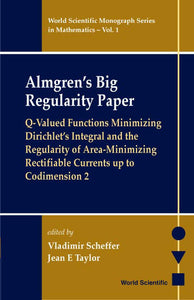# Almgren's Big Regularity Paper, Q-valued Functions Minimizing Dirichlet's Integral And The Regularit

Format: Print Book

ISBN: 9789810241087

• \$156.81
Unit price per
Tax included.

Fred Almgren exploited the excess method for proving regularity theorems in the calculus of variations. His techniques yielded Hölder continuous differentiability except for a small closed singular set. In the sixties and seventies Almgren refined and generalized his methods. Between 1974 and 1984 he wrote a 1,700-page proof that was his most ambitious development of his ground-breaking ideas. Originally, this monograph was available only as a three-volume work of limited circulation. The entire text is faithfully reproduced here.This book gives a complete proof of the interior regularity of an area-minimizing rectifiable current up to Hausdorff codimension 2. The argument uses the theory of Q-valued functions, which is developed in detail. For example, this work shows how first variation estimates from squash and squeeze deformations yield a monotonicity theorem for the normalized frequency of oscillation of a Q-valued function that minimizes a generalized Dirichlet integral. The principal features of the book include an extension theorem analogous to Kirszbraun's theorem and theorems on the approximation in mass of nearly flat mass-minimizing rectifiable currents by graphs and images of Lipschitz Q-valued functions.

Format: Hardcover
No of Pages: 972
Imprint: World Scientific
Publication date: 20000703
Series: World Scientific Monograph Series In Mathematics